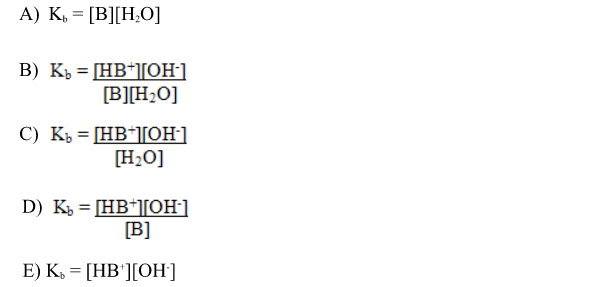Chemistry Practice Problems Equilibrium Expressions Practice Problems Solution: Which of the following is the correct K b expressi...

🤓 Based on our data, we think this question is relevant for Professor Upadhyaya's class at USF.

# Solution: Which of the following is the correct K b expression for the reaction below:B(aq) + H2O(l) ⇌ HB+ (aq) + OH– (aq)

###### Problem

Which of the following is the correct K b expression for the reaction below:

B(aq) + H2O(l) ⇌ HB+ (aq) + OH (aq)Equilibrium Expressions

Equilibrium Expressions

#### Q. When the following reaction is at equilibrium, which of these relationships is  always true?2 NOCl (g)  ⇋ 2 NO (g) + Cl 2 (g)

Solved • Wed Mar 22 2017 12:41:06 GMT-0400 (EDT)

Equilibrium Expressions

#### Q. At a certain temperature, K c equals 1.4 × 10 2 for the reaction:2 CO (g) + O 2 (g) ⇌ 2 CO 2 (g).If a 2.50-L flask contains 0.400 mol of CO 2 and 0.10...

Solved • Wed Mar 22 2017 11:45:03 GMT-0400 (EDT)

Equilibrium Expressions

#### Q. What is the equilibrium  constant, Kc, for an equilibrium mixture for the reaction2 BrCl3(g) ⇋ Br2(g) + 3 Cl2(g)which contains 1.0 mol BrCl3, 2.0 mol ...

Solved • Wed Mar 22 2017 11:23:07 GMT-0400 (EDT)

Equilibrium Expressions

#### Q. Write the equilibrium expression, Kc, for the following chemical reaction equation.2 C6H6(g) + 15 O2(g) ⇋ 12 CO2(g) + 6 H2O(g)

Solved • Wed Mar 22 2017 11:09:41 GMT-0400 (EDT)